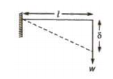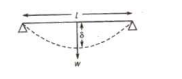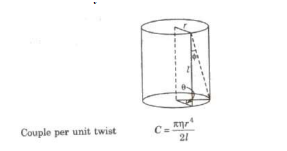# Mechanical Properties of Solids class 11 Notes Physics

CBSE class 11 Physics Chapter 9 Mechanical Properties of Solids  class 11 Notes Physics in PDF are available for free download in myCBSEguide mobile app. The best app for CBSE students now provides Mechanical Properties of Solids class 11 Notes Physics latest chapter wise notes for quick preparation of CBSE exams and school based annual examinations.  on Chapter 9 Mechanical Properties of Solids class 11 Notes Physics  are also available for download in CBSE Guide website.

## CBSE Guide Mechanical Properties of Solids class 11 Notes

CBSE guide notes are the comprehensive notes which covers the latest syllabus of CBSE and NCERT. It includes all the topics given in NCERT class 11 Physics text book. Users can download CBSE guide quick revision notes from myCBSEguide mobile app and my CBSE guide website.

## 11 Physics notes Chapter 9 Mechanical Properties of Solids

Download CBSE class 11th revision notes for Chapter 9 Mechanical Properties of Solids class 11 Notes Physics in PDF format for free. Download revision notes for Mechanical Properties of Solids class 11 Notes Physics and score high in exams. These are the Mechanical Properties of Solids class 11 Notes Physics prepared by team of expert teachers. The revision notes help you revise the whole chapter in minutes. Revising notes in exam days is on of the the best tips recommended by teachers during exam days.

CBSE Class XI PHYSICS
Revision Notes
CHAPTER 9
MECHANICAL PROPERTIES OF SOLIDS

• Elastic behaviour of solids
• Stress and strain
• Hooke’s law
• Young’s modulus, bulk modulus, shear modulus of rigidity
• Poisson’s Ratio, Elastic Energy

Deforming Force : A force which produces a change in configuration of the object on applying it, is called a deforming force.

Elasticity: Elasticity is that property of the object by virtue of which it regain its original configuration after the removal of the deforming force.

Elastic Limit  : Elastic limit is the upper limit of deforming force upto which, if deforming force is removed, the body regains its original form completely and beyond which if deforming force is increased the body loses its property of elasticity and get permanently deformed.

Perfectly Elastic Bodies: Those bodies which regain its original configuration immediately and completely after the removal of deforming force are called perfectly elastic bodies. e.g., quartz and phosphor bronze etc.

Perfectly Plastic Bodies: Those bodies which does not regain its original configuration at all on the removal of deforming force are called perfectly plastic bodies, e.g., putty, paraffin, wax etc.

Stress : The internal restoring force acting per unit area of a deformed body is called stress.

Stress =

Its unit is N/m2 or Pascal and dimensional formula is[].

Stress is a tensor quantity.

Stress is of Two Types

(i) Normal Stress If deforming force is applied normal to the area, then the stress is called normal stress.

If there is an increase in length, then stress is called tensile stress.

If there is a decrease in length, then stress is called compression stress.

(ii) Tangential Stress If deforming force is applied tangentially, then the stress is called tangential stress.

Strain : The fractional change in configuration is called strain.

Strain =

It has no unit and it is a dimensionless quantity. According to the change in configuration, the strain is of three types

(1) Longitudinal strain=

(2) Volumetric strain =

(iii) Shearing strain = Angular displacement of the plane perpendicular to the fixed surface.

Hooke’s Law

Within the limit of elasticity, the stress is proportional to the strain.

Stress &infi; Strain

or Stress = E * Strain

where, E is the modulus of elasticity of the material of the body.

Types of Modulus of Elasticity

1. Young’s Modulus of Elasticity

It is defined as the ratio of normal stress to the longitudinal strain Within the elastic limit.

y = Normal stress / Longitudinal strain

Its unit is N/m2 or Pascal and its dimensional formula is [].

2. Bulk Modulus of Elasticity

It is defined as the ratio of normal stress to the volumetric strain within the elastic limit.

K =

K = = Δp V / Δ V

where, Δp = Change in pressure.

Its unit is N/m2 or Pascal and its dimensional formula is [M-1T-2 ].

3. Modulus of Rigidity (η)

It is defined as the ratio of tangential stress to the shearing strain, within the elastic limit.

η = Tangential stress / Shearing strain

Its urut is N/m2 or Pascal and its dimensional formula is [ML-1T-2 ].

Compressibility

Compressibility of a material is the reciprocal of its bulk modulus of elasticity. Compressibility (C) = 1 / k

Its SI unit is N-1m2 and CGS unit is dyne-1 cm2 . Steel is more elastic than rubber. Solids are more elastic and gases are least elastic. For liquids. modulus of rigidity is zero. Young’s modulus (Y) and modulus of rigidity (η) are possessed by solid materials only.

Limit of Elasticity

The maximum value of deforming force for which elasticity is present in the body is called its limit of elasticity.

Breaking Stress

The minimum value of stress required to break a wire, is called breaking stress. Breaking stress is fixed for a material but breaking force varies with area of cross-section of the wire. Safety factor =

Elastic Relaxation Time

The time delay in restoring the original configuration after removal of deforming force is called elastic relaxation time.

For quartz and phosphor bronze this time is negligible.

Elastic After Effect

The temporary delay in regaining the original configuration by the elastic body after the removal of deforming force, is called elastic after effect.

Elastic Fatigue

The property of an elastic body by virtue of which its behaviour becomes less elastic under the action of repeated alternating deforming force is called elastic fatigue.

Ductile Materials

The materials which show large plastic range beyond elastic limit are called ductile materials, e.g., copper, silver, iron, aluminum, etc. Ductile materials are used for making springs and sheets. Brittle Materials The materials which show very small plastic range beyond elastic limit are called brittle materials, e.g., glass, cast iron, etc.

Elastomers

The materials for which strain produced is much larger than the stress applied, with in the limit of elasticity are called elastomers, e.g., rubber, the elastic tissue of aorta, the large vessel carrying blood from heart. etc. Elastomers have no plastic range.

Elastic Potential Energy in a Stretched Wire

The work done in stretching a wire is stored in form of potential energy of the wire.

Potential energy U = Average force * Increase in length

= Stress * Strain * Volume of the wire

Elastic potential energy per unit volume

U = * Stress * Strain

=  (Young’s modulus) * (Strain)2

Elastic potential energy of a stretched spring = kx2

where, k = Force constant of spring and x = Change in length.

Thermal Stress When temperature of a rod fixed at its both ends is changed, then the produced stress is called thermal stress.

Thermal stress =  = yαΔθ

where, α = coefficient of linear expansion of the material of the rod.

When temperature of a gas enclosed in a vessel is changed, then the thermal stress produced is equal to change in pressure (Δp)of the gas.

Thermal stress = Δ p = Ky Δ θ

where, K = bulk modulus of elasticity and

γ = coefficient of cubical expansion of the gas.

Interatomic force constant

K = Yro

where, ro = interatomic distance.

Poisson’s Ratio

When a deforming force is applied at the free end of a suspended wire of length 1 and radius R, then its length increases by dl but its radius decreases by dR. Now two types of strains are produced by a single force.

1. Longitudinal strain = ΔUl
2. ∴ Poisson’s Ratio (σ) =  = The theoretical value of Poisson’s ratio lies between – 1 and 0.5. Its practical value lies between 0 and 0.5

Relation Between Y, K, η and σ

(i) Y = 3K (1 – 2σ)

(ii) Y = 2 η ( 1 + σ)

Coefficient of elasticity depends upon the material, its temperature and purity but not on stress or strain.

For the same material, the three coefficients of elasticity γ, η and K have different magnitudes.

Isothermal elasticity of a gas ET = ρ where, ρ = pressure of the gas.

Adiabatic elasticity of a gas Es = γρ where, γ = ;  ratio of specific heats at constant pressure and at constant volume

Ratio between isothermal elasticity and adiabatic elasticity;

Cantilever
A beam clamped at one end and loaded at free end is called a cantilever. Depression at the free end of a cantilever is given by

δ =where, w = load, 1 = length of the cantilever,
y = Young’s modulus of elasticity, and  = geometrical moment of inertia.
For a beam of rectangular cross-section having breadth b and thickness d.

For a beam of circular cross-section area having radius r

Beam Supported at Two Ends and Loaded at the MiddleDepression at middle δ =

Torsion of a Cylinderwhere, η = modulus of rigidity of the material of cylinder, r = radius of cylinder,
and 1 = length of cylinder,
Work done in twisting the cylinder through an angle θ
W = 1 / 2 Cθ2
Relation between angle of twist (θ) and angle of shear (φ) rθ = lφ or φ = r / l = θ

## Mechanical Properties of Solids class 11 Notes

• CBSE Revision notes for Class 11 Physics PDF
• CBSE Revision notes Class 11 Physics – CBSE
• CBSE Revisions notes and Key Points Class 11 Physics
• Summary of the NCERT books all chapters in Physics class 11
• Short notes for CBSE class 11th Physics
• Key notes and chapter summary of Physics class 11
• Quick revision notes for CBSE exams

## CBSE Class-11 Revision Notes and Key Points

Mechanical Properties of Solids  class 11 Notes Physics. CBSE quick revision note for class-11 Physics, Chemistry, Maths, Biology and other subject are very helpful to revise the whole syllabus during exam days. The revision notes covers all important formulas and concepts given in the chapter. Even if you wish to have an overview of a chapter, quick revision notes are here to do if for you. These notes will certainly save your time during stressful exam days.

To download Mechanical Properties of Solids class 11 Notes Physics, sample paper for class 11 Physics, Chemistry, Biology, History, Political Science, Economics, Geography, Computer Science, Home Science, Accountancy, Business Studies and Home Science; do check myCBSEguide app or website. myCBSEguide provides sample papers with solution, test papers for chapter-wise practice, NCERT solutions, NCERT Exemplar solutions, quick revision notes for ready reference, CBSE guess papers and CBSE important question papers. Sample Paper all are made available through the best app for CBSE students and myCBSEguide website.Test Generator

Create question paper PDF and online tests with your own name & logo in minutes.myCBSEguide

Question Bank, Mock Tests, Exam Papers, NCERT Solutions, Sample Papers, Notes